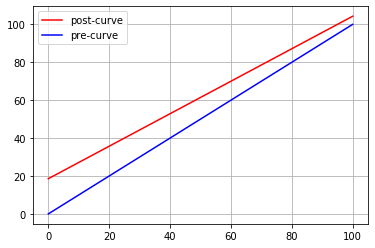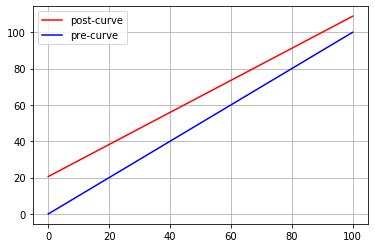# On "Curving" Exam Scores¶

I sometimes curve exam scores using a linear scale of the general form $f(x) = mx + b$, where $x$ is the score to be curved.

To compute the parameters of the linear formula, I first calculate the average score on the exam, $\bar{x}$, and decide on an appropriate "perfect" score $x_p$ (which is often the maximum earned score, though it may also be different to mitigate "curve busters"). The job of the scaling formula is to map $\bar{x}$ to the desired average (typically 70%), and $x_p$ onto 100%. The following formula accomplishes this:

$$f(x) = 70 + \frac{100 - 70}{x_p - \bar{x}}(x-\bar{x})$$

For example, if the average score on an exam is 60%, and the chosen perfect score is 95%, this gives us the equation:

$$f(x) = 70 + \frac{100 - 70}{95 - 60}(x-60) = 70 + \frac{30}{35}(x-60)$$

The cell below shows the result of applying this specific curve to a variety of different values:

In :
def f(x):
return 70 + (30/35)*(x-60)

[(x, f(x)) for x in range(40, 101, 5)]

Out:
[(40, 52.85714285714286),
(45, 57.142857142857146),
(50, 61.42857142857143),
(55, 65.71428571428571),
(60, 70.0),
(65, 74.28571428571429),
(70, 78.57142857142857),
(75, 82.85714285714286),
(80, 87.14285714285714),
(85, 91.42857142857143),
(90, 95.71428571428571),
(95, 100.0),
(100, 104.28571428571428)]

Note that the formula will raise all scores, but will give an extra boost to lower scores. Scores above the "perfect score" will result in curved scores > 100%.

The following cell plots scores pre and post curve, based on this formula.

In :
%matplotlib inline
import matplotlib.pyplot as plt
import numpy as np

def plot_curve(xm, xp):
x = np.linspace(0, 100, 100)
y = (70+(30/(xp-xm))*(x-xm))
plt.plot(x, y, color='red', label='post-curve')
plt.plot(x, x, color='blue', label='pre-curve')
plt.legend(loc='upper left')
plt.grid()
plt.show()

plot_curve(60, 95)## Midterm 1¶

The average on midterm 1 was 56%, and I determined 90% to be a reasonable "perfect" score (only two students scored higher than this). This gives us the following formula to compute the curves score:

$$f(x) = 70 + \frac{100 - 70}{90 - 56}(x-56) = 70 + \frac{30}{34}(x-56)$$
In :
def midterm1(x):
return 70 + (30/34)*(x-56)

[(x, f(x)) for x in range(40, 101, 5)]

Out:
[(40, 52.85714285714286),
(45, 57.142857142857146),
(50, 61.42857142857143),
(55, 65.71428571428571),
(60, 70.0),
(65, 74.28571428571429),
(70, 78.57142857142857),
(75, 82.85714285714286),
(80, 87.14285714285714),
(85, 91.42857142857143),
(90, 95.71428571428571),
(95, 100.0),
(100, 104.28571428571428)]

Here's the corresponding plot:

In :
plot_curve(56, 90)